# Basic Examples on Functions

Go back to  'Functions'

Let us quickly go through some trivial examples so that you start getting familiar with the whole idea of functions:

Example- 1

The graph of a function f is shown in Figure 6.

(a) Find the values of f (1) and f(5)

(b) What are the domain and range of f ?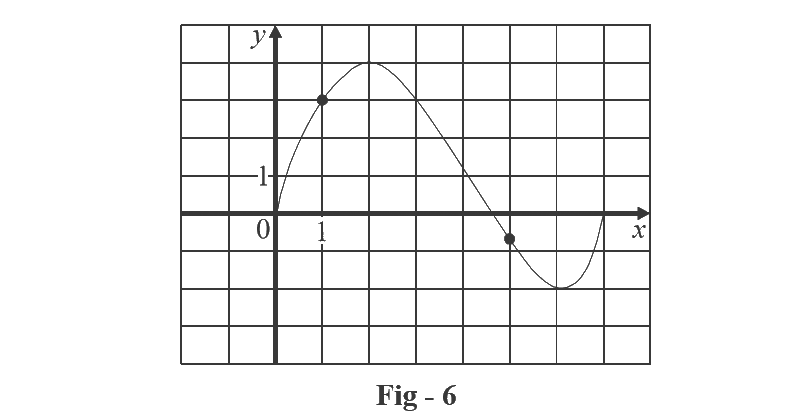Solution:

(a) We see from Figure - 6 that the point (1,3) lies on the graph of f, so the value of f at 1 is f (1) = 3. (In other words, the point on the graph that lies above x = 1 is 3 units above the x -axis.)

When x = 5, the graph lies about 0.7 unit below the x-axis, so we estimate that $$f\left( 5 \right) \approx - 0.7$$.

(b) We see that $$f\left( x \right)$$ is defined when $$0 \le x \le 7$$, so the domain of f is the closed interval [0, 7]. Notice that f takes on all values from –2 to 4, so the range of f is

$\left\{ {y|-2 \le y \le 4} \right\} = \left[ { - 2,4} \right]$

Example- 2

Sketch the graph and find the domain and range of each function.

(a) $$f\left( x \right) = 2x - 1$$

(b) $$g\left( x \right) = {x^2}$$

Solution: (a) The equation of the graph is $$y = 2x - 1$$, and we recognize this as being the equation of a line with slope 2 and y–intercept –1. (Recall the slope - intercept form of the equation of a line: $$y = mx + c$$ ). This enables us to sketch the graph of f in figure 7. The expression $$2x - 1$$ is defined for all real numbers, so the domain of f is the set of all real numbers, which we denote by $$\mathbb{R}$$. The graph shows that the range is also $$\mathbb{R}$$.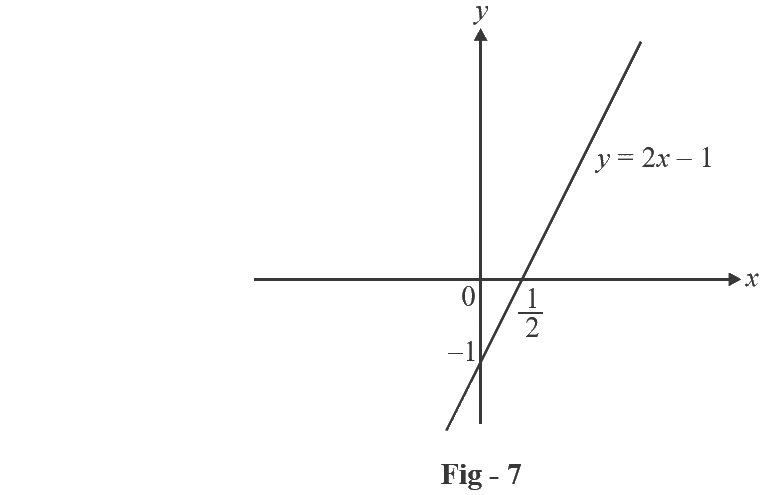(b) Since $$g\left( 2 \right) = {2^2} = 4$$ and $$g\left( { - 1} \right) = {\left( { - 1} \right)^2} = 1$$, we could plot the points (2, 4) and (–1, 1), together with a few other points on the graph, and join them to produce the graph (figure 8). The equation of the graph is $$y = {x^2}$$, which represents something called a parabola. The domain of g is $$\mathbb{R}$$. The range of g consists of all values of $$g\left(x \right)$$, that is, all numbers of the form $${x^2}$$. But $${x^2} \ge 0$$ for all numbers x and any positive number y is a square, so the range of g is $$\left\{ {y\,|\,y \ge 0} \right\} = \left[ {0,\infty } \right)$$. This can also be seen from Figure 8.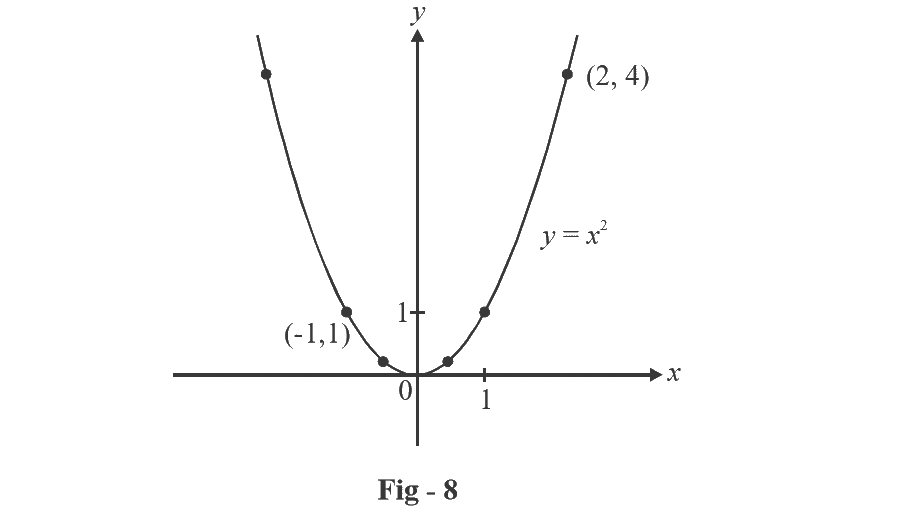Sometimes, the domain may be specified explicitly, like this:

$$f\left( x \right) = {x^2},\qquad\qquad x \in \left[ {2,3} \right]$$

So, although $${x^2}$$ is defined for all real values of x, in this particular case, we will consider it only on the interval specified, that is, on $$\left[ {2,3} \right]\,$$.

Likewise, the range depends on the domain:

$$f\left( x \right) = {x^2}$$          has a range of $$\left[ {0,\infty } \right)$$

$$f\left( x \right) = {x^2}, \qquad x \in \left[ {2,3} \right]$$ has a range of $$\left[ {4,9} \right]$$

$$f\left( x \right) = {x^2}, \qquad x \in \left\{ {2,3} \right\}$$ has a range of $$\left\{ {4,9} \right\}$$ etc.

There are four possible ways to represent a function:

verbally           (by a description in words)

numerically     (by a table of values)

visually            (by a graph)

algebraically    (by an explicit formula)

If a single function can be represented in all four ways, it is often useful to go from one representation to another to gain additional insight into the function. (In Example 2, for instance, we started with algebraic formulas and then obtain the graphs.) But certain functions are described more naturally by one method than by another. With this in mind, let’s re-examine two of four situations that we considered at the beginning of this section.

(a) The most useful representation of the area of a circle as a function of its radius is probably the algebraic formula $$A\left( r \right) = \pi {r^2}$$, though it is possible to compile a table of values or to sketch a graph (half a parabola). Because a circle has to have a positive radius, the domain is $$\left\{ {r|r > 0} \right\} = \left( {0,\infty } \right)$$, and the range is also $$\left( {0,\infty } \right)$$.

(b) We are given a description of the function in words: $$P\left( t \right)$$ is the human population of the world at time t. If we plot these values from some standard table of values of world population, we get the graph (called a scatter plot ) in figure 9. It too is useful representation; the graph allows us to absorb all the data at once. What about a formula? Of course, it’s impossible to devise an explicit formula that gives the exact human population $$P\left( t \right)\,$$ at any time. But it is possible to find an expression for a function that approximates $$P\left( t \right)$$. In fact, using certain methods you’ll come across later, we obtain the approximation

$P\left( t \right) \approx f\left( t \right) = \left( {0.008079266} \right).{\left( {1.013731} \right)^t}$

and Figure 10 shows that it is a reasonably good “fit.” The function f is called a mathematical model for population growth. In other words, it is a function with an explicit formula that approximates the behavior of our given function. We will see, however, that the ideas of calculus can be applied to approximate values; an explicit formula is not necessary.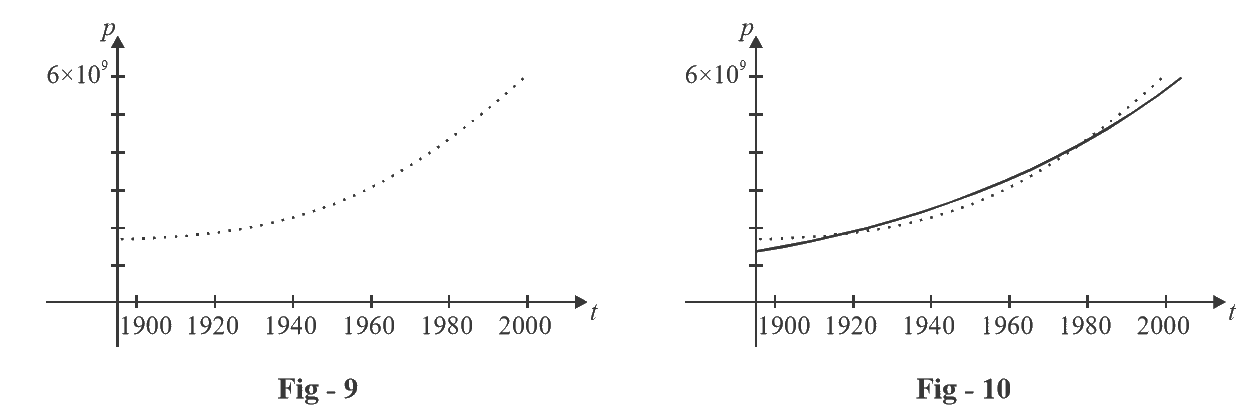The function P is typical of the functions that arise whenever we attempt to apply calculus to the real world. We start with a verbal description of a function. Then we may be able to construct a table of values of the function, perhaps from instrument readings in a scientific experiment. Even though we don’t have complete knowledge of the values of the function, we will see throughout our study of calculus that it is still possible to perform the operations of calculus on such a function.

In the next example we sketch the graph of a function that is defined verbally.

Example- 3

When you turn on a hot - water faucet, the temperature T of the water depends on how long the water has been running. Draw a rough graph of T as a function of the time t that has elapsed since the faucet was turned on.

Solution: The initial temperature of the running water is close to room temperature because of the water that has been sitting in the pipes. When the water from the hot - water tank starts coming out, T increases quickly. In the next phase, T is constant at the temperature of the heated water in the tank. When the tank is drained, T decreases to the temperature of the water supply. This enables us to make a rough sketch of T as a function of t in figure 11.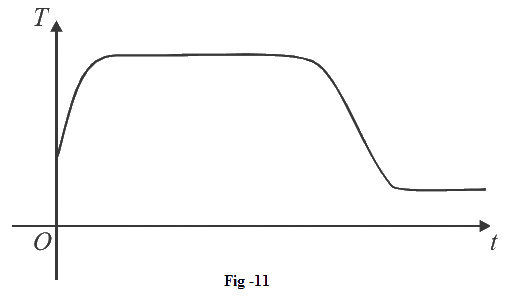In the following example we start with a verbal description of a function in a physical situation and obtain an explicit algebraic formula. The ability to do this is a useful skill in solving calculus problems that ask for the maximum or minimum values of quantities.

Example- 4

A rectangular storage container with an open top has a volume of 10 m3. The length of its base is twice its width. Material for the base costs Rs 10 per square meter; material for the sides costs Rs 6 per square meter. Express the cost of materials as a function of the width of the base.

Solution: We draw a diagram as in Figure 12 and introduce notation by letting w and 2w be the width and length of the base, respectively, and h be the height.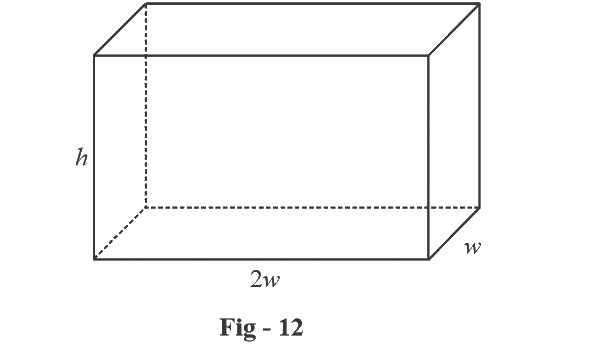The area of the base is $$\left( {2w} \right)w = 2{w^2}$$, so the cost, in rupees of the material for the base is $$10\left( {2{w^2}} \right)$$. Two of the sides have area wh and the other two have area 2wh, so the cost of the material for the sides is $$6\left[ {2\left( {wh} \right) + 2\left( {2wh} \right)} \right]$$. The total cost is therefore

$C = 10\left( {2{w^2}} \right) + 6\left[ {2\left( {wh} \right) + 2\left( {2wh} \right)} \right] = 20{w^2} + 36wh$

To express C as a function of w alone, we need to eliminate h and we do so by using the fact that volume is 10 m3. Thus

$w\left( {2w} \right)h = 10$

which gives

\begin{align}&h = \frac{{10}}{{2{w^2}}} = \frac{5}{{{w^2}}}\end{align}

Substituting this into the expression for C, we have

\begin{align}&C = 20{w^2} + 36w\left( {\frac{5}{{{w^2}}}} \right) = 20{w^2} + \frac{{180}}{w}\end{align}

Therefore, the equation

\begin{align}&C\left( w \right) = 20{w^2} + \frac{{180}}{w} w > 0\end{align}

expresses C as a function of w.